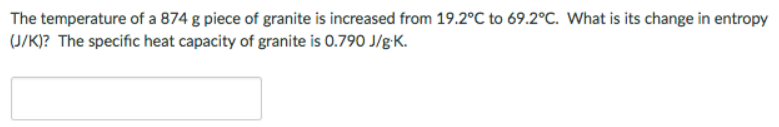Chemistry Practice Problems Entropy Practice Problems Solution: The temperature of a 874 g piece of granite is inc...

# Solution: The temperature of a 874 g piece of granite is increased from 19.2°C to 69.2°C. What is its change in entropy J/K)? The specific heat capacity of granite is 0.790 J/g • K.

###### Problem

The temperature of a 874 g piece of granite is increased from 19.2°C to 69.2°C. What is its change in entropy J/K)? The specific heat capacity of granite is 0.790 J/g • K.View Complete Written Solution

Entropy

Entropy

#### Q. The entropy of 0.002 mole of CH2CI2 (aq) is greater than the entropy of 0.002 mole of CH2CI2 (l).a. Trueb. False

Solved • Tue Jul 24 2018 10:25:41 GMT-0400 (EDT)

Entropy

#### Q. The entropy of 0.5 mole LiBr (s) at 290 K is greater than the entropy of 0.5 mole LiBr (s) at 330Ka. Trueb. False

Solved • Tue Jul 24 2018 10:17:29 GMT-0400 (EDT)

Entropy

#### Q. A 15 g piece of metal (138.4 g/mol) melts at 1774 °C and its enthalpy of fusion is 17.4/mol. Calculate the entropy of fusion (J/K) of the metal. Enter...

Solved • Tue Jul 03 2018 13:12:00 GMT-0400 (EDT)

Entropy

#### Q. Consider the reaction: I2 (g) + Cl2 (g) → 2ICl (g) Using standard absolute entropies at 298K, calculate the entropy change for the system when 2.49 mo...

Solved • Tue Jul 03 2018 11:35:33 GMT-0400 (EDT)